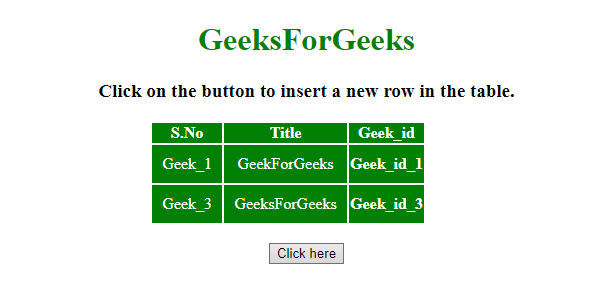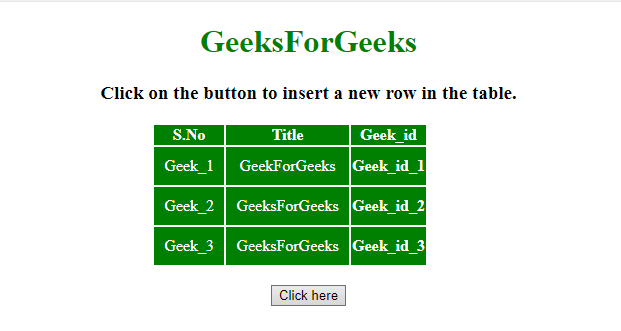# How to insert new row at a certain index in a table in jQuery ?

Given an HTML document containing a table. The task is to insert a new row in that table at a certain index using JQuery.

Approach:

• Store the table column value <td> element into the variable.
• Then use eq() and after() method to insert the row in a table.

Example 1: In this example, the row is inserted at index 1 (hardcoded).

 `  ` `<``html``>  ` `    ``<``head``>  ` `        ``<``title``>  ` `            ``Insert new row at a certain index ` `            ``in a table using jQuery ` `        ``  ` `         `  `        ``<``script` `src` `=  ` `"https://ajax.googleapis.com/ajax/libs/jquery/3.4.0/jquery.min.js"``> ` `        `` ` `         `  `        ``<``style``> ` `            ``#myCol { ` `                ``background:green; ` `            ``} ` `            ``table { ` `                ``color:white; ` `            ``} ` `            ``#Geek_p { ` `                ``color:green; ` `                ``font-size:30px; ` `            ``} ` `            ``td { ` `                ``padding:10px; ` `            ``} ` `        `` ` `    `` ` `     `  `    ``<``body``>  ` `        ``<``center``>     ` `        ``<``h1` `style` `= ``"color:green;"` `>  ` `            ``GeeksForGeeks  ` `        ``  ` `         `  `        ``<``strong``> ` `            ``Click on the button to insert ` `            ``a new row in the table ` `        `` ` `         `  `        ``<``br``><``br``> ` `         `  `        ``<``table``> ` `            ``<``colgroup``> ` `                ``<``col` `id``=``"myCol"` `                    ``span``=``"2"``> ` `                ``<``col` `style``=``"background-color:green"``> ` `            `` ` `             `  `            ``<``tr``> ` `                ``<``th``>S.No ` `                ``<``th``>Title ` `                ``<``th``>Geek_id ` `            `` ` `            ``<``tr` `id` `= ``"row1"``> ` `                ``<``td``>Geek_1 ` `                ``<``td``>GeekForGeeks ` `                ``<``th``>Geek_id_1 ` `            `` ` `            ``<``tr``> ` `                ``<``td``>Geek_3 ` `                ``<``td``>GeeksForGeeks ` `                ``<``th``>Geek_id_3 ` `            `` ` `        `` ` `        ``<``br``> ` `         `  `        ``<``button` `onclick` `= ``"Geeks()"``>  ` `            ``Click here ` `        ``  ` `         `  `        ``` `        ``<``script``>  ` `            ``function Geeks() { ` `                ``var html =  ` `                ``"<``td``>Geek_2<``td``>GeeksForGeeks<``th``>Geek_id_2"; ` `                 `  `                ``\$('table > tbody > tr').eq(1).after(html); ` `            ``} ` `        ``  ` `        `` ` `    ``  ` `                     `

Output:

• Before clicking on the button:• After clicking on the button:Example 2: In this example, the row is inserted at index provided by the user.

 `  ` `<``html``>  ` `    ``<``head``>  ` `        ``<``title``>  ` `            ``Insert new row at a certain index ` `            ``in a table using jQuery ` `        ``  ` `         `  `        ``<``script` `src` `=  ` `"https://ajax.googleapis.com/ajax/libs/jquery/3.4.0/jquery.min.js"``> ` `        `` ` `         `  `        ``<``style``> ` `            ``#myCol { ` `                ``background:green; ` `            ``} ` `            ``table { ` `                ``color:white; ` `            ``} ` `            ``#Geek_p { ` `                ``color:green; ` `                ``font-size:30px; ` `            ``} ` `            ``td { ` `                ``padding:10px; ` `            ``} ` `        `` ` `    `` ` `     `  `    ``<``body``>  ` `        ``<``center``>     ` `        ``<``h1` `style` `= ``"color:green;"` `>  ` `            ``GeeksForGeeks  ` `        ``  ` `         `  `        ``<``strong``> ` `            ``Click on the button to insert ` `            ``a new row in the table ` `        `` ` `         `  `        ``<``br``><``br``> ` `         `  `        ``<``table``> ` `            ``<``colgroup``> ` `                ``<``col` `id``=``"myCol"` `                    ``span``=``"2"``> ` `                ``<``col` `style``=``"background-color:green"``> ` `            `` ` `             `  `            ``<``tr``> ` `                ``<``th``>S.No ` `                ``<``th``>Title ` `                ``<``th``>Geek_id ` `            `` ` `            ``<``tr` `id` `= ``"row1"``> ` `                ``<``td``>Geek_1 ` `                ``<``td``>GeekForGeeks ` `                ``<``th``>Geek_id_1 ` `            `` ` `            ``<``tr``> ` `                ``<``td``>Geek_3 ` `                ``<``td``>GeeksForGeeks ` `                ``<``th``>Geek_id_3 ` `            `` ` `        `` ` `        ``<``br``> ` `         `  `        ``<``button` `onclick` `= ``"Geeks()"``>  ` `            ``Click here ` `        ``  ` `         `  `        ``` `        ``<``script``>  ` `            ``function Geeks() { ` `                ``var i = 2; ` `                ``var html =  ` `                ``"<``td``>Geek_2<``td``>GeeksForGeeks<``th``>Geek_id_2"; ` `                 `  `                ``\$('table > tbody > tr').eq(i - 1).after(html); ` `            ``} ` `        ``  ` `        `` ` `    ``  ` `                     `

Output:

• Before clicking on the button:• After clicking on the button:Whether you're preparing for your first job interview or aiming to upskill in this ever-evolving tech landscape, GeeksforGeeks Courses are your key to success. We provide top-quality content at affordable prices, all geared towards accelerating your growth in a time-bound manner. Join the millions we've already empowered, and we're here to do the same for you. Don't miss out - check it out now!

Previous
Next